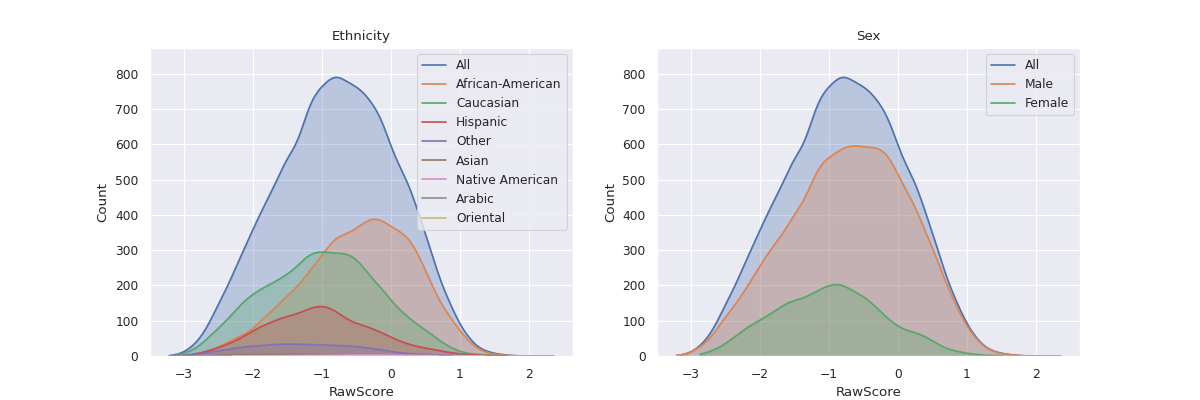# fairlens.plot.mult_distr_plot#

mult_distr_plot(df, target_attr, attrs, figsize=None, max_width=3, distr_type=None, attr_distr_types=None, max_quantiles=8, show_hist=None, show_curve=None, shade=True, normalize=False, cmap=None)[source]#

Plot the distribution of the all values for each of the unique values in the column attr with respect to the target attribute.

Parameters
• df (pd.DataFrame) – The input dataframe.

• target_attr (str) – The target attribute.

• attrs (Sequence[str]) – The attributes whose value distributions are to be plotted.

• figsize (Optional[Tuple[int, int]], optional) – The size of each figure if separate is True. Defaults to (6, 4).

• max_width (int, optional) – The maximum amount of figures. Defaults to 3.

• distr_type (Optional[str], optional) – The type of distribution of the target attribute. Can take values from [“categorical”, “continuous”, “binary”, “datetime”]. If None, the type of distribution is inferred based on the data in the column. Defaults to None.

• attr_distr_types (Optional[Mapping[str, str]], optional) – The types of distribution of the attributes in attrs. Passed as a mapping from attribute name to corresponding distribution type. Can take values from [“categorical”, “continuous”, “binary”, “datetime”]. If None, the type of distribution of all sensitive attributes are inferred based on the data in the respective columns. Defaults to None.

• max_quantiles (int, optional) – The maximum amount of quantiles to use for continuous data. Defaults to 8.

• show_hist (Optional[bool], optional) – Shows the histogram if True. Defaults to True if the data is categorical or binary.

• show_curve (Optional[bool], optional) – Shows a KDE if True. Defaults to True if the data is continuous or a date.

• shade (bool, optional) – Shades the curve if True. Defaults to True.

• normalize (bool, optional) – Normalizes the counts so the sum of the bar heights is 1. Defaults to False.

• cmap (Optional[Sequence[Tuple[float, float, float]]], optional) – A sequence of RGB tuples used to colour the histograms. If None seaborn’s default pallete will be used. Defaults to None.

Examples

```>>> df = pd.read_csv("datasets/compas.csv")
>>> mult_distr_plot(df, "RawScore", ["Ethnicity", "Sex", "MaritalStatus", "Language", "DateOfBirth"])
>>> plt.show()
```